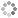# PROWAREtecharticles » current » dot-net » check-number-for-power-of-2

## .NET: Is a Number a Power of Two (2)

How to check or determine if a number has a base of 2 without using modulo operations for maximum performance; written in C#.

See how to find a number with a base of 2 in JavaScript.

### Solution One: Use log2

The simplest way to determine if a number is a power of two is to use log2 and it will return an integer if so.

### Solution Two: Bit-wise Operations

Bit-wise operations are very fast and this should be the preferred method.

If the number is a power of two then the number AND (&) the same number minus one (1) will not be zero (0) unless the number itself is zero.

``````
bool isBase2(int num)
{
return (num & (num - 1)) == 0 && num != 0;
}
``````

Here is the above function in a working example.

``````
namespace ConsoleApp1
{
class Program
{
static bool isBase2(int num)
{
return (num & (num - 1)) == 0 && num != 0;
}

static void Main(string[] args)
{
System.Console.WriteLine("0=" + isBase2(0) + " 1=" + isBase2(1) + " 2=" + isBase2(2) + " 3=" + isBase2(3) + " 4=" + isBase2(4) + " 10=" + isBase2(10) + " 12=" + isBase2(12) + " 16=" + isBase2(16) + " 32=" + isBase2(32) + " 33=" + isBase2(33));
}
}
}
``````This site uses cookies. Cookies are simple text files stored on the user's computer. They are used for adding features and security to this site. Read the privacy policy.